10. Wearables¶

Inspired by my last project, the

VAGINATECH¶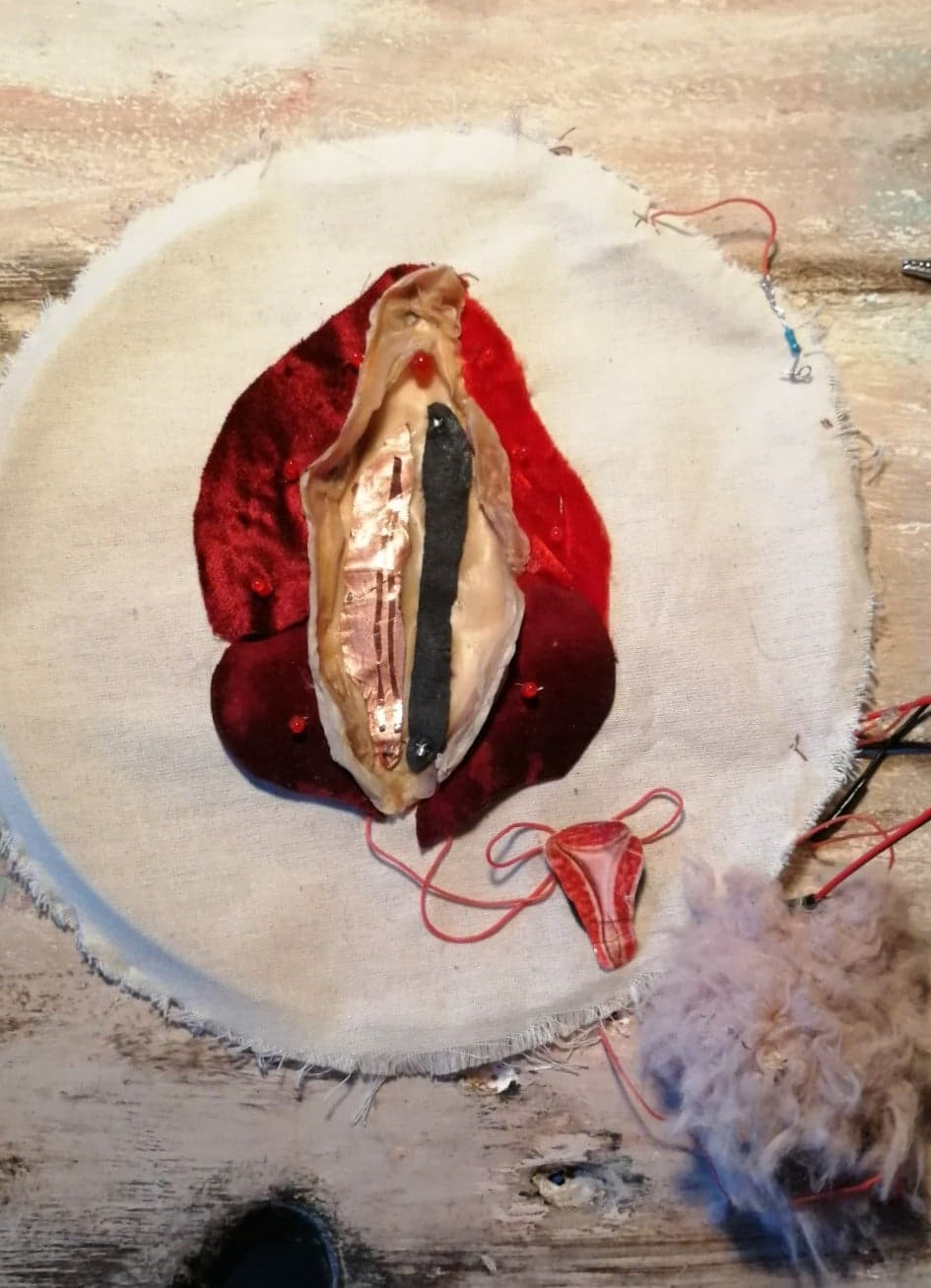I mixed sound and female power and create the

SYNTHUTERUS¶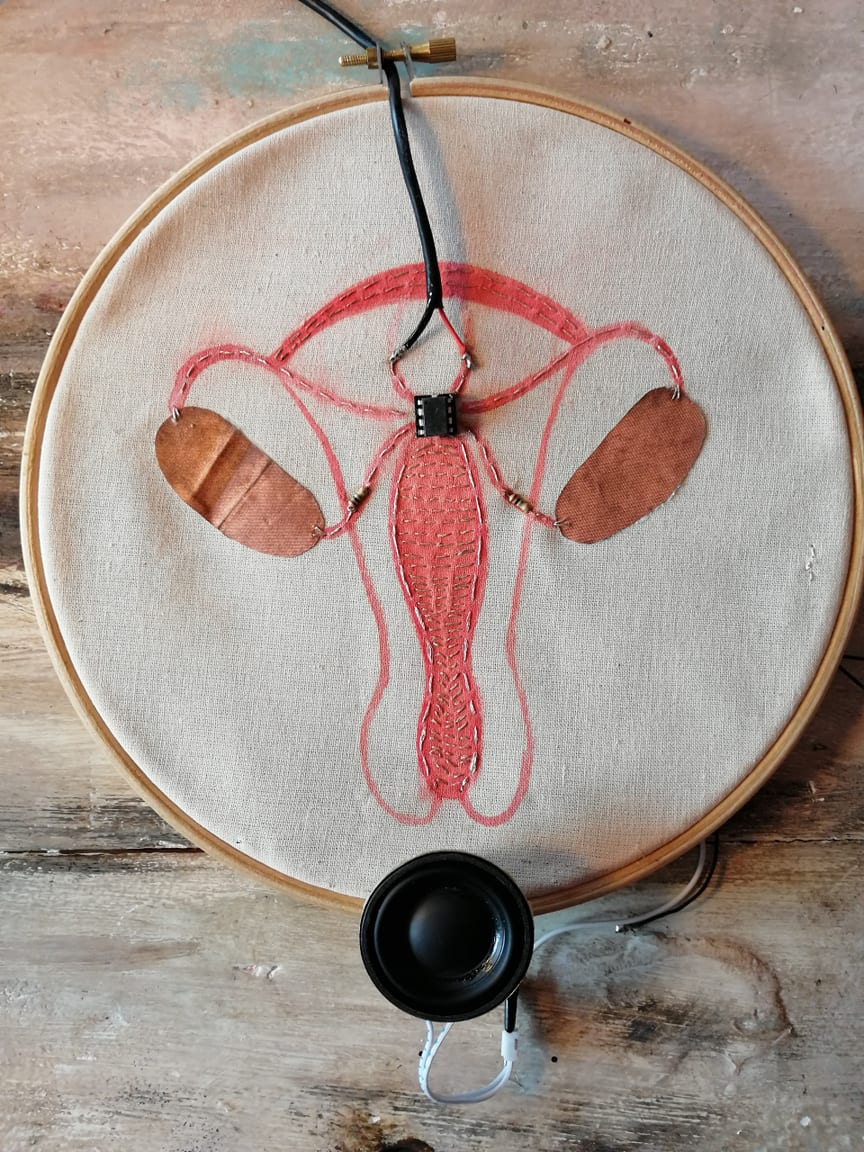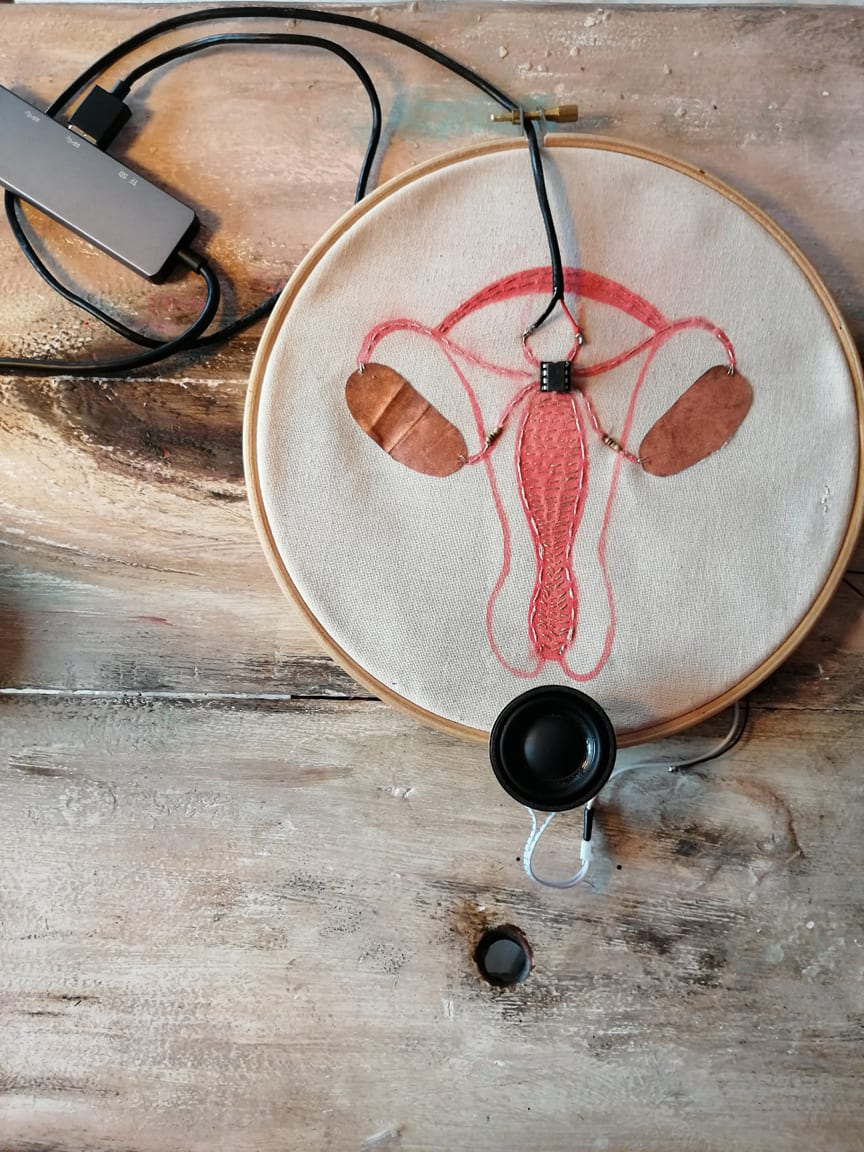Silicon speakers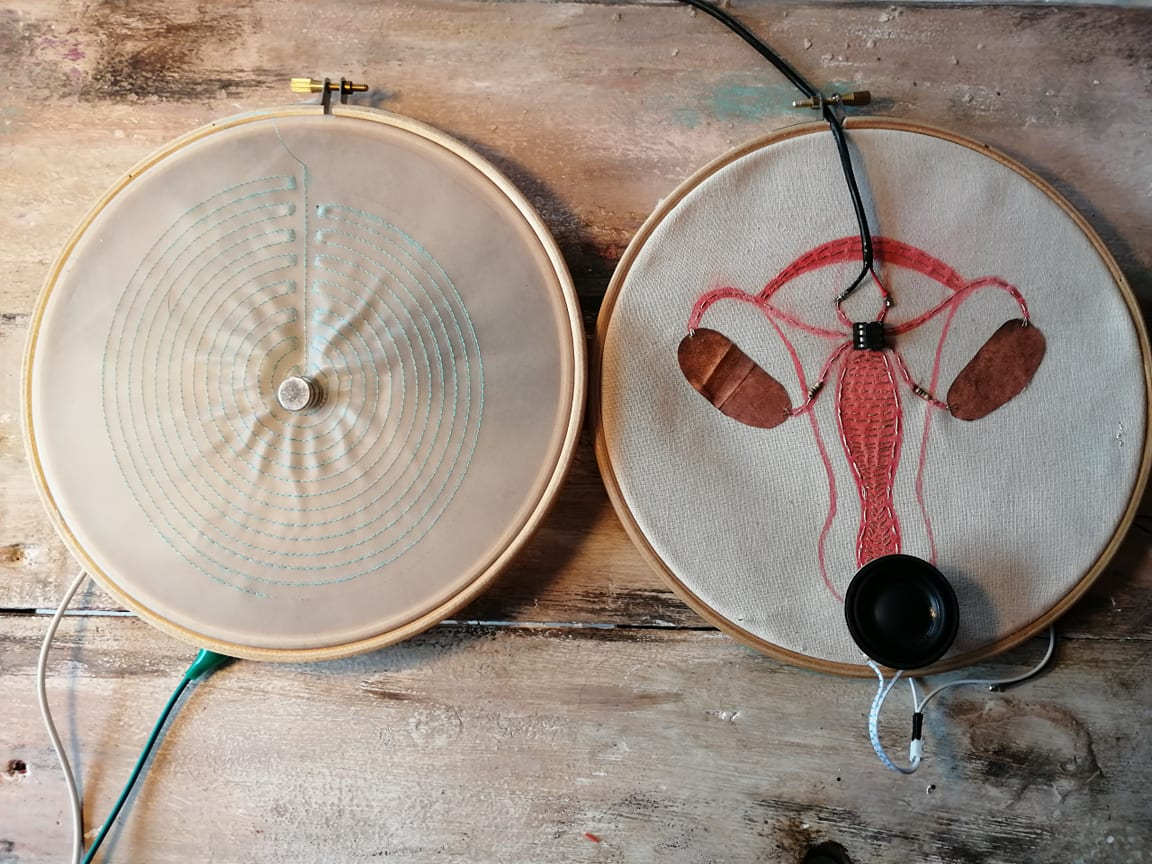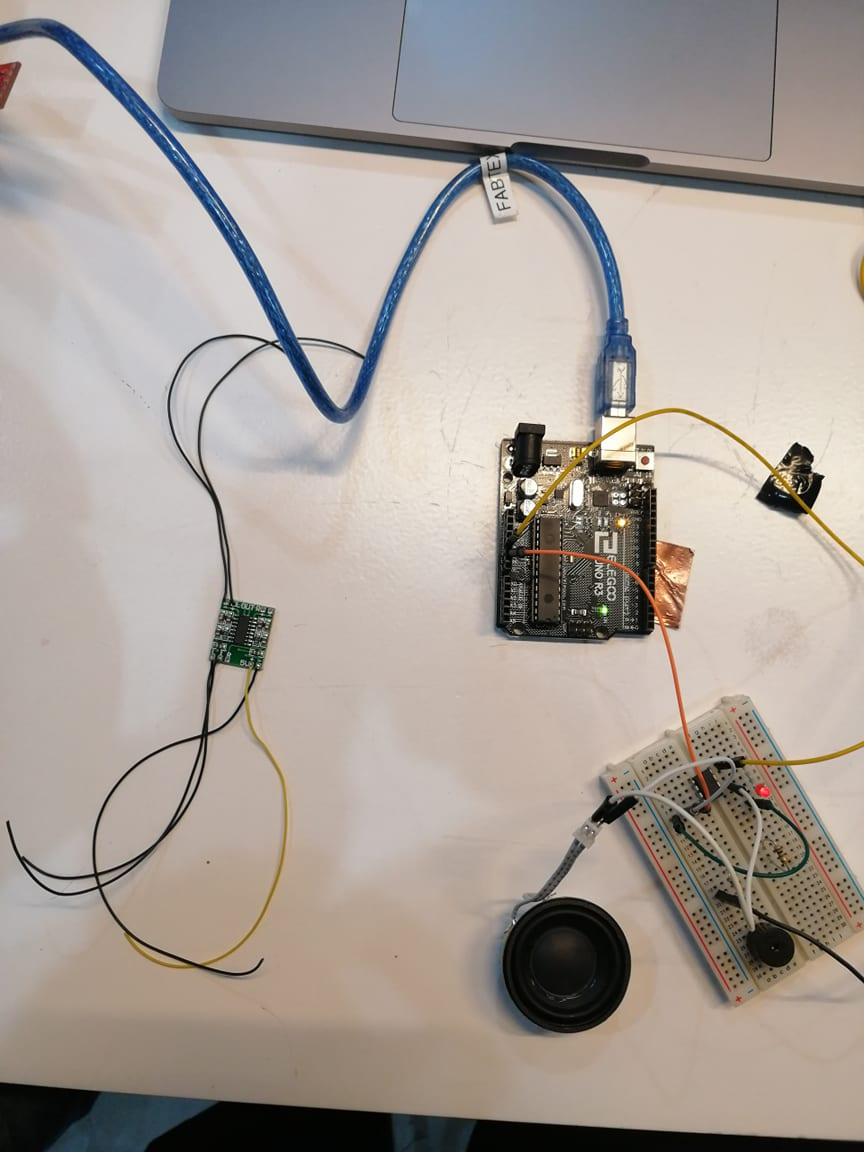Code Example AtTiny¶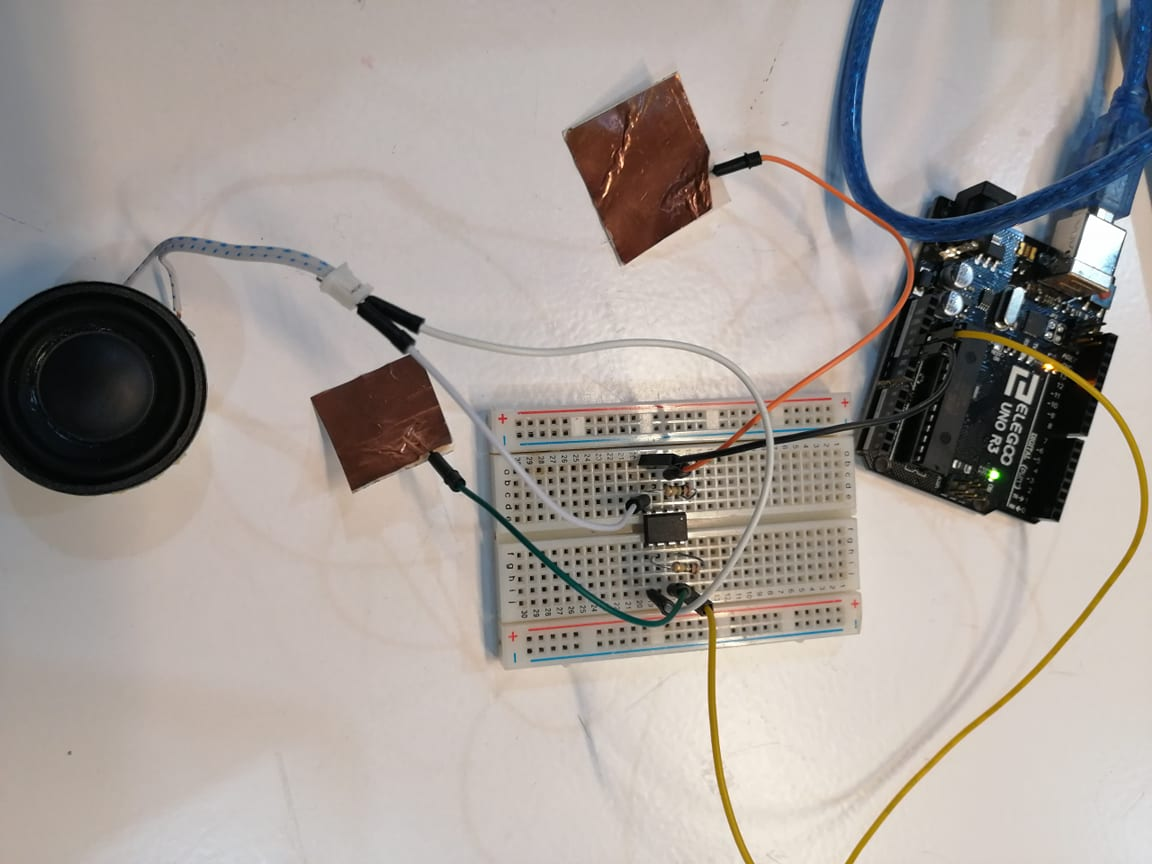/*
* chainStitch Noise for ATtiny85
*
* Two capacitive sensors controlling a white noise generator.
*
* https://en.wikipedia.org/wiki/Linear_feedback_shift_register
* http://www.electricdruid.net/index.php?page=techniques.practicalLFSRs
*
* Afroditi Psarra
* June 2016
*/

#include <CapacitiveSensor.h> // Use the capacitive sensing library.

CapacitiveSensor right = CapacitiveSensor(1, 2); // 1MOhms resistor between digital pin 1 and 2, 2 is the sensor pin.
CapacitiveSensor left = CapacitiveSensor(4, 3); // 1MOhms resistor between digital pin 4 and 3, 3 is the sensor pin.

long rightVal = 0; // Variable to store the right sensor's value
int freqVal = 0; // Variable to store the mapped right sensor's value - to be later used as frequency modulator

long leftVal = 0; // Variable to store the left sensor's value
int outVal = 0; // Variable to store the mapped left sensor's value

int speakerPin = 0; // Speaker connected to digital pin 0

unsigned long int reg;

void setup() {
pinMode(speakerPin, OUTPUT); // initialize the speakerPin as an output
reg = 0x55aa55aaL; //The seed for the bitstream. It can be anything except 0.
}

void loop() {
rightVal = right.capacitiveSensor(30); // read the right sensor's value
freqVal = map(rightVal, 0, 50000, 500, 0); // map the right sensor's value in a frequency range to be used as frequency modulation.

leftVal = left.capacitiveSensor(30); // read the left sensor's value
outVal = map(leftVal, 0, 50000, 40, 0); // map the left sensor's value in milliseconds to be used as a delay between each grain repetition.

if (rightVal > 40) {
for (int i = 0; i < 150; i++) {
generateNoise();
}
delay(outVal);
}

if (leftVal > 40) {
for (int i = 0; i < 500; i++) {
generateNoise();
}
delay(outVal);
}

else {
digitalWrite(speakerPin, LOW);
}
}

// the noise producing function
void generateNoise() {
unsigned long int newr;
unsigned char lobit;
unsigned char b31, b29, b25, b24;
b31 = (reg & (1L << 31)) >> 31;
b29 = (reg & (1L << 29)) >> 29;
b25 = (reg & (1L << 25)) >> 25;
b24 = (reg & (1L << 24)) >> 24;
lobit = b31 ^ b29 ^ b25 ^ b24;
newr = (reg << 1) | lobit;
reg = newr;
digitalWrite(speakerPin, reg & 1);

delayMicroseconds(freqVal); // Changing this value changes the frequency.
}

Last update: December 1, 2020